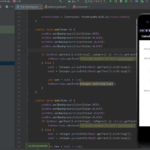## Simple Calculator In Android With Source Code

The Simple Calculator is a simple beginners level Android project. It functions fully as similar to a normal calculator. Here you can perform all of your calculations with ease. To run the project you will need Android Studio. So before you run the projec...

Type : Project

File Size : 10.4 MB# Similar Projects and Reports

#### Simple Calculator In Android With Source Code

The Simple Calculator is a simple beginners level Android project. It functions fully as similar to a normal calculator. Here you can perform all of your calculations with ease. To run the project you will need Android Studio. So before you run the projec...

#### Calculator App In Android With Source Code

The Calculator app is a simple Android project. It functions fully as similar to a normal calculator. Here you can perform all of your calculations with ease. To run the project you will need Android Studio. So before you run the project make sure that yo...

#### Simple Calculator In C# With Source Code

Simple Calculator in C# is based on the concept of normal mathematical operations on the calculator. The project contains the numbers, operators, and signs like in a normal calculator. So, the user cannot enter the number themselves, they can just click o...

#### SIMPLE CALCULATOR IN PYTHON WITH SOURCE CODE

The Simple Calculator In Python is a simple project developed using Python. The project contains the numbers, operators, and sign like in normal calculator. The user can enter any number or can just click on the numbers they want in the calculations. Henc...

#### SIMPLE BMI CALCULATOR IN PHP WITH SOURCE CODE

BMI Calculator is a simple project developed using HTML5, CSS, and PHP. This project is for checking your BMI. With the BMI value, you can check whether you have a healthy weight or not. Also, you can see the basic features of this calculator in this proj...

#### Simple Interest Calculator In C++ With Source code

The Simple Interest Calculator is based on the concept of mathematical calculations of loan amount. This whole system is in the C++ language. The project is for user convenience, to help them find out about the monthly payments on a specific loan. This pr...